# 相位反冲

## 1. 探索CNOT门

CNOT\ket{0+} = \tfrac{1}{\sqrt{2}}(\ket{00} + \ket{11})

from qiskit import QuantumCircuit, Aer, assemble
from math import pi
import numpy as np
from qiskit.visualization import plot_bloch_multivector, plot_histogram, array_to_latex

qc = QuantumCircuit(2)
qc.h(0)
qc.h(1)
qc.cx(0,1)
qc.draw()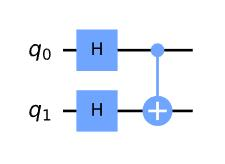\ket{++} = \tfrac{1}{\sqrt{2}}(\ket{00} + \ket{01} + \ket{10} + \ket{11})

qc = QuantumCircuit(2)
qc.h(0)
qc.h(1)
qc.cx(0,1)
display(qc.draw())  # display is a command for Jupyter notebooks
# similar to print, but for rich content

# Let's see the result
svsim = Aer.get_backend('aer_simulator')
qc.save_statevector()
qobj = assemble(qc)
final_state = svsim.run(qobj).result().get_statevector()
display(array_to_latex(final_state, prefix="\\text{Statevector} = "))
plot_bloch_multivector(final_state)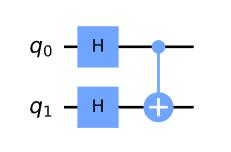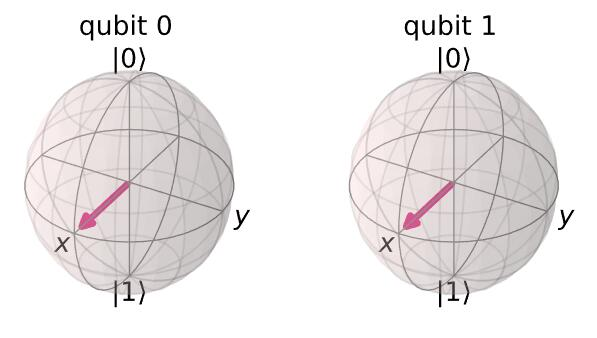qc = QuantumCircuit(2)
qc.h(0)
qc.x(1)
qc.h(1)
qc.draw()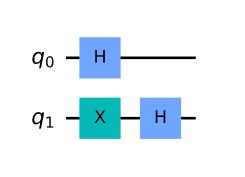\ket{-+} = \tfrac{1}{\sqrt{2}}(\ket{00} + \ket{01} - \ket{10} - \ket{11})
qc = QuantumCircuit(2)
qc.h(0)
qc.x(1)
qc.h(1)
display(qc.draw())
# See the result
qc1 = qc.copy()
qc1.save_statevector()
final_state = svsim.run(qc1).result().get_statevector()
display(array_to_latex(final_state, prefix="\\text{Statevector} = "))
plot_bloch_multivector(final_state)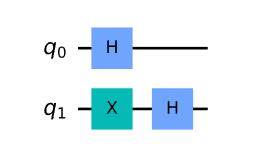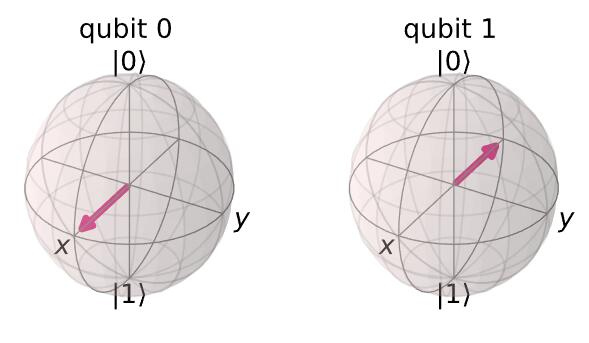\begin{aligned} CNOT\ket{-+} & = \tfrac{1}{2}(\ket{00} - \ket{01} - \ket{10} + \ket{11}) \\ & = \ket{--} \end{aligned}

qc.cx(0,1)
display(qc.draw())

qc.save_statevector()
qobj = assemble(qc)
final_state = svsim.run(qobj).result().get_statevector()
display(array_to_latex(final_state, prefix="\\text{Statevector} = "))
plot_bloch_multivector(final_state)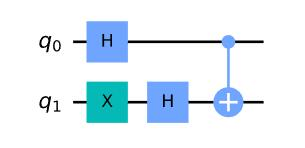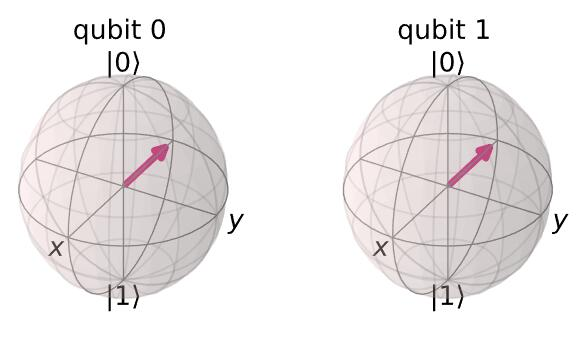qc = QuantumCircuit(2)
qc.h(0)
qc.h(1)
qc.cx(0,1)
qc.h(0)
qc.h(1)
display(qc.draw())

qc.save_unitary()
usim = Aer.get_backend('aer_simulator')
qobj = assemble(qc)
unitary = usim.run(qobj).result().get_unitary()
array_to_latex(unitary, prefix="\\text{Circuit = }\n")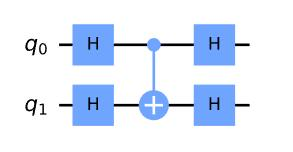qc = QuantumCircuit(2)
qc.cx(1,0)
display(qc.draw())
qc.save_unitary()

qobj = assemble(qc)
unitary = usim.run(qobj).result().get_unitary()
array_to_latex(unitary, prefix="\\text{Circuit = }\n")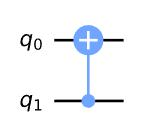## 2. 相位反冲

### 2.1 解释CNOT电路的特性X\ket{-} = -\ket{-}

\begin{aligned} CNOT\ket{-0} & = \ket{-} \otimes \ket{0} \\ & = \ket{-0} \\ \quad & \\ CNOT\ket{-1} & = X\ket{-} \otimes \ket{1} \\ & = -\ket{-} \otimes \ket{1} \\ & = -\ket{-1} \\ \end{aligned}

\begin{aligned} CNOT\ket{-+} & = \tfrac{1}{\sqrt{2}}(CNOT\ket{-0} + CNOT\ket{-1}) \\ & = \tfrac{1}{\sqrt{2}}(\ket{-0} + X\ket{-1}) \\ & = \tfrac{1}{\sqrt{2}}(\ket{-0} - \ket{-1}) \\ \end{aligned}

\begin{aligned} CNOT\ket{-+} & = \tfrac{1}{\sqrt{2}}(\ket{0} - \ket{1}) \\ & = \ket{--} \end{aligned}

### 2.2 T门的相位反冲

qc = QuantumCircuit(2)
qc.cp(pi/4, 0, 1)
qc.draw()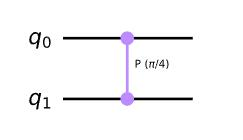T门的矩阵为：

T = \begin{bmatrix} 1 & 0 \\ 0 & e^{i\pi/4}\\ \end{bmatrix}

Controlled-T = \begin{bmatrix} 1 & 0 & 0 & 0 \\ 0 & 1 & 0 & 0 \\ 0 & 0 & 1 & 0 \\ 0 & 0 & 0 & e^{i\pi/4}\\ \end{bmatrix}

qc = QuantumCircuit(2)
qc.cp(pi/4, 0, 1)
display(qc.draw())
# See Results:
qc.save_unitary()
qobj = assemble(qc)
unitary = usim.run(qobj).result().get_unitary()
array_to_latex(unitary, prefix="\\text{Controlled-T} = \n")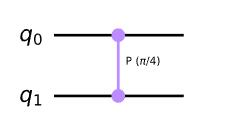Controlled-T = \begin{bmatrix} 1 & 0 & 0 & 0 \\ 0 & 1 & 0 & 0 \\ 0 & 0 & 1 & 0 \\ 0 & 0 & 0 & \tfrac{1}{\sqrt{2}}(1 + i) \\ \end{bmatrix}

\begin{aligned} U = \begin{bmatrix} u_{00} & u_{01} \\ u_{10} & u_{11} \\ \end{bmatrix} \end{aligned}
\begin{aligned} Controlled-U = \begin{bmatrix} I & 0 \\ 0 & U \\ \end{bmatrix} = \begin{bmatrix} 1 & 0 & 0 & 0 \\ 0 & 1 & 0 & 0 \\ 0 & 0 & u_{00} & u_{01} \\ 0 & 0 & u_{10} & u_{11}\\ \end{bmatrix} \end{aligned}

Controlled-U = \begin{bmatrix} 1 & 0 & 0 & 0 \\ 0 & u_{00} & 0 & u_{01} \\ 0 & 0 & 1 & 0 \\ 0 & u_{10} & 0 & u_{11}\\ \end{bmatrix}

T\ket{1} = e^{i\pi/4}\ket{1}

\begin{aligned} \ket{1+} & = \ket{1} \otimes \tfrac{1}{\sqrt{2}}(\ket{0} + \ket{1}) \\ & = \tfrac{1}{\sqrt{2}}(\ket{10} + \ket{11}) \\ & \\ Controlled-T\ket{1+} & = \tfrac{1}{\sqrt{2}}(\ket{10} + e^{i\pi/4}\ket{11}) \\ & \\ & = \ket{1} \otimes \tfrac{1}{\sqrt{2}}(\ket{0} + e^{i\pi/4}\ket{1}) \end{aligned}

qc = QuantumCircuit(2)
qc.h(0)
qc.x(1)
display(qc.draw())
# See Results:
qc.save_statevector()
qobj = assemble(qc)
final_state = svsim.run(qobj).result().get_statevector()
plot_bloch_multivector(final_state)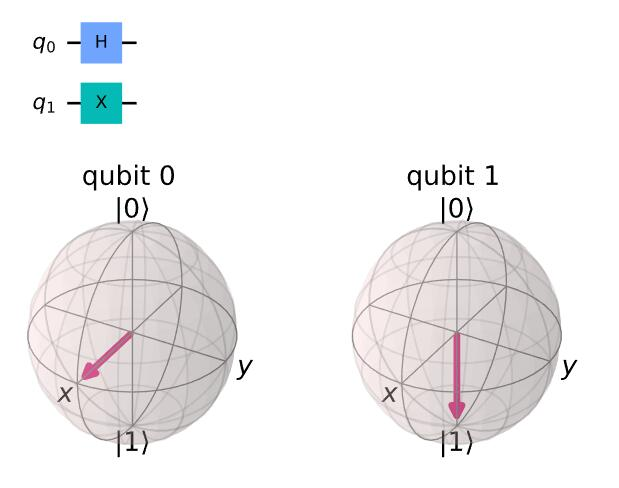qc = QuantumCircuit(2)
qc.h(0)
qc.x(1)
qc.cp(pi/4, 0, 1)
display(qc.draw())
# See Results:
qc.save_statevector()
qobj = assemble(qc)
final_state = svsim.run(qobj).result().get_statevector()
plot_bloch_multivector(final_state)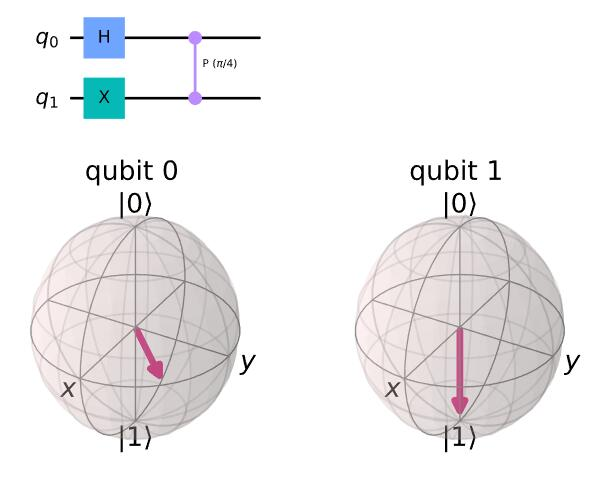### 快速练习

1. 如果目标量子比特(q1)处于状态 \ket{0} ，那么控制量子比特(q0)的最终状态是什么？（如下图所示电路）？用Qiskit来验证你的答案。2. 如果目标量子比特(q1)处于状态 \ket{1} ，且电路使用受控Sdg门而不是受控T（如下图所示电路），控制量子比特(q0)会发生什么变化？3. 在应用受控T（如下图所示电路）之前，如果控制量子比特(q0)处于状态 \ket{1} ，而不是状态 \ket{+} ，将会发生什么变化?import qiskit.tools.jupyter
%qiskit_version_table


### 版本信息

Qiskit Software Version
Qiskit 0.27.0
Terra 0.17.4
Aer 0.8.2
Ignis 0.6.0
Aqua 0.9.2
IBM Q Provider 0.14.0
System information
Python 3.7.7 (default, May 6 2020, 04:59:01) [Clang 4.0.1 (tags/RELEASE_401/final)]
OS Darwin
CPUs 8
Memory (Gb) 32.0

Fri Jun 18 18:38:49 2021 BST